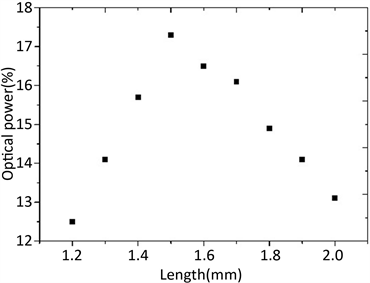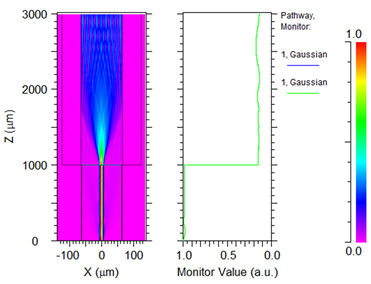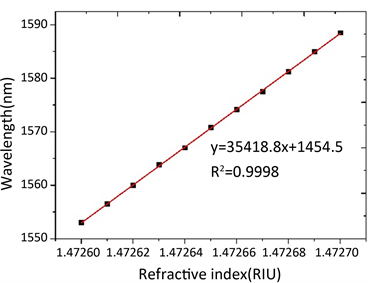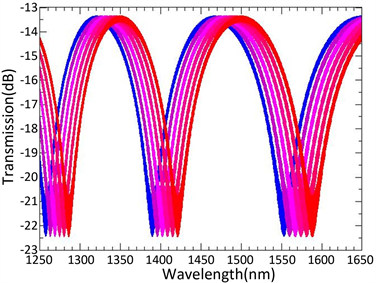﻿ 基于光纤迈克尔逊干涉仪的食用油折射率的快速测量

基于光纤迈克尔逊干涉仪的食用油折射率的快速测量Rapid Analysis of Edible-Oil Refractive Index Based on Fiber Optical Michelson Interferometer

Abstract: Refractive index is an important indicator of edible oil quality. In this paper, we proposed a rapid measurement method for the refractive index of edible oil based on a new fiber Michelson interferometry structure. It combined a glass capillary with the classical dual-arm fiber optical Michelson interferometer. The simulation results confirmed that this method could quickly measure the refractive index of edible oil, and has a high sensitivity. The resolution is lower than 0.000001. This method provides a convenient and effective way for testing edible oil quality.

1. 引言

2. 测量装置及原理

${I}_{1}=q\sqrt{{r}_{1}}I$ (1)

${I}_{2}=\left(1-q\right)\sqrt{{r}_{2}}I{\text{e}}^{-j2{\varphi }_{1}},\text{\hspace{0.17em}}\text{\hspace{0.17em}}{\varphi }_{1}=\frac{2\text{π}{n}_{1}\left({l}_{2}-{l}_{1}\right)}{\lambda }$ (2)

${I}_{3}=\left(1-q\right)\left(1-{r}_{2}\right)\sqrt{{r}_{3}}{\alpha }^{2}I{\text{e}}^{-j2{\varphi }_{2}},\text{\hspace{0.17em}}\text{\hspace{0.17em}}{\varphi }_{2}=\frac{2\text{π}\left({n}_{1}{l}_{2}+{n}_{2}{l}_{3}-{n}_{1}{l}_{1}\right)}{\lambda }$ (3)

${r}_{1}=\frac{{\left({n}_{1}-1\right)}^{2}}{{\left({n}_{1}+1\right)}^{2}}$ (4)

${r}_{2}=\frac{{\left({n}_{1}-{n}_{2}\right)}^{2}}{{\left({n}_{1}+{n}_{2}\right)}^{2}}$ (5)

$\begin{array}{c}{I}_{4}=|\frac{{I}_{1}+{I}_{2}+{I}_{3}}{I}|\\ ={q}^{2}{r}_{1}+{\left(1-q\right)}^{2}{r}_{2}^{2}+{\left(1-q\right)}^{2}{\left(1-{r}_{2}\right)}^{2}{r}_{3}^{2}+q\left(1-q\right)\sqrt{{r}_{1}}\sqrt{{r}_{2}}\mathrm{cos}\left(2{\varphi }_{1}\right)\\ \text{\hspace{0.17em}}\text{\hspace{0.17em}}+q\left(1-q\right)\left(1-{r}_{2}\right)\sqrt{{r}_{1}}\sqrt{{r}_{3}}\mathrm{cos}\left(2{\varphi }_{2}\right)+{\left(1-q\right)}^{2}\left(1-{r}_{2}\right)\sqrt{{r}_{2}}\sqrt{{r}_{3}}\mathrm{cos}\left(2{\varphi }_{1}-2{\varphi }_{2}\right)\end{array}$ (6)

$a={n}_{1}{l}_{2}+{n}_{2}{l}_{3}-{n}_{1}{l}_{1}$ (7)

${\lambda }_{N}=\frac{{n}_{1}{l}_{2}+{n}_{2}{l}_{3}-{n}_{1}{l}_{1}}{2N+1},\text{\hspace{0.17em}}\text{\hspace{0.17em}}N=0,1,2,3,\cdots$ (8)

3. 模拟分析(a)(b)

Figure 3. (a) Beam propagation in the glass capillary filled with oil; (b) optical power change with the length of the glass capillary(a)(b)

Figure 4. (a) Beam propagation in the glass capillary filled with oil; (b) optical power change with the length of the glass capillary

4. 结论

 黄伟, 郑建军, 徐建华. 地沟油的安全快速检测研究[J]. 山东科技大学学报, 2010, 29(3): 51-53.

 胡欣宇. 基于电导率法的地沟油含量测定[J]. 物联网技术, 2017, 7(5): 26-31.

 杨洁, 黄海深, 李阳军. 基于互补金属氧化物半导体的高精度地沟油检测计[J]. 食品与机械, 2016, 32(9): 44-48.

 Li, J.S. (2010) Optical Parameters of Vegetable Oil Studied by Terahertz Time-Domain Spectroscopy. Applied Spectroscopy, 64, 231-234.
https://doi.org/10.1366/000370210790619663

 Wu, Z., Li, H. and Tu, D.W. (2015) Application of Fourier Transform Infrared (FT-IR) Spectroscopy Combined with Chemo Metrics for Analysis of Rapeseed Oil Adulterated with Refining and Purificating Waste Cooking Oil. Food Analytical Methods, 8, 2581-2587.
https://doi.org/10.1007/s12161-015-0149-z

 李昊, 陈明惠. 荧光光谱分析法在地沟油鉴别中的应用研究[J]. 激光生物学报, 2014, 23(5): 472-476.

 张丙芳, 苑立波, 孔庆明, 等. 近红外光谱技术快速鉴别地沟油与使用植物油的研究[J]. 光谱学与光谱分析, 2014, 34(10): 2723-2727.

 庄俊域, 冯志强, 林丹, 等. 综合指标评价餐厨废油脂的研究[J]. 食品工业科技, 2014, 35(14): 127-130.

 Fukuta, M., Yanagisawa, T., Miyamura, S. and Ogi, Y. (2004) Concentration Measurement of Refrigerant/Refrigeration Oil Mixture by Refractive Index. International Journal of Refrigeration, 27, 346-352.

 石亚新, 葛武鹏, 吴小勇, 等. 地沟油甄别检测技术研究进展[J]. 食品科学, 2016, 37(7): 276-281.

 花世群, 骆英, 洪云. 基于等厚干涉原理的液体折射率测量方法[J]. 中国激光, 2006, 33(11): 1542-1546.

 张浩, 陈明惠, 田甜, 项华中, 郑刚. 食用油折射率的光干涉快速测量方法[J]. 光学仪器, 2017, 39(1): 1-5.

 姚建永, 张森, 王臻, 周琦, 刘孟华. 光纤迈克尔逊干涉仪的理论与应用分析[J]. 仪表技术与传感器, 2007(5): 23-25.

Top